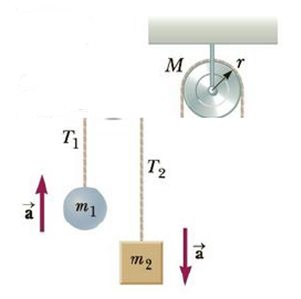Chapter 8, Problem 46P

Chapter
Section
Textbook Problem

An Atwood’s machine consists of blocks of masses m1 = 10.0 kg and m2 = 20.0 kg attached by a cord running over a pulley as in Figure P8.46. The pulley is a solid cylinder with mass M = 8.00 kg and radius r = 0.200 m. The block of mass m2 is allowed to drop, and the cord turns the pulley without slipping, (a) Why must the tension T2 be greater than the tension T1? (b) What is the acceleration of the system, assuming the pulley axis is frictionless? (c) Find the tensions T1, and T2.Figure P8.46

(a)

To determine

Why the tension T2 should be greater than T1 ?

Explanation

The figures shows that the system will accelerate in all direction,

The tensions T1 and T2 can’t be equal because there should be a net torque in order to have angular acceleration

(b)

To determine

The acceleration of the system.

(c)

To determine

The tensions T1 and T2 .

Still sussing out bartleby?

Check out a sample textbook solution.

See a sample solution

The Solution to Your Study Problems

Bartleby provides explanations to thousands of textbook problems written by our experts, many with advanced degrees!

Get Started